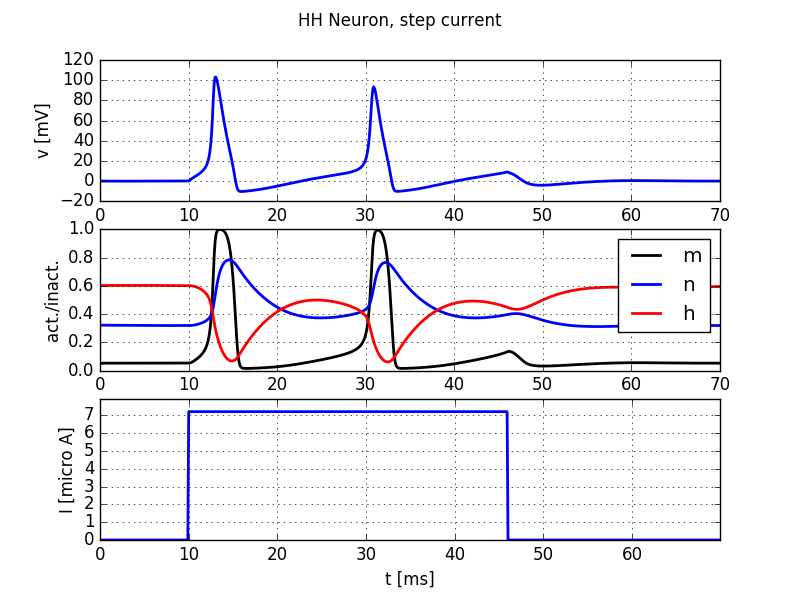# 6. Numerical integration of the HH model of the squid axon¶

Book chapters

See Chapter 2 Section 2 on general information about the Hodgkin-Huxley equations and models.

Python classes

The hodgkin_huxley.HH module contains all code required for this exercise. It implements a Hodgkin-Huxley neuron model. At the beginning of your exercise solutions, import the modules and run the demo function.

%matplotlib inline  # needed in Jupyter notebooks, not in Python scripts.
import brian2 as b2
import matplotlib.pyplot as plt
import numpy as np
from neurodynex.hodgkin_huxley import HH
from neurodynex.tools import input_factory

HH.getting_started()Step current injection into a Hodgkin-Huxley neuron

## 6.1. Exercise: step current response¶

We study the response of a Hodgkin-Huxley neuron to different input currents. Have a look at the documentation of the functions HH.simulate_HH_neuron() and HH.plot_data() and the module neurodynex.tools.input_factory.

### 6.1.1. Question¶

What is the lowest step current amplitude I_min for generating at least one spike? Determine the value by trying different input amplitudes in the code fragment:

current = input_factory.get_step_current(5, 100, b2.ms, I_min *b2.uA)
state_monitor = HH.simulate_HH_neuron(current, 120 * b2.ms)
HH.plot_data(state_monitor, title="HH Neuron, minimal current")


### 6.1.2. Question¶

What is the lowest step current amplitude to generate repetitive firing?

## 6.2. Exercise: slow and fast ramp current¶

The minimal current to elicit a spike does not just depend on the amplitude I or on the total charge Q of the current, but on the “shape” of the current. Let’s see why:

### 6.2.1. Question¶

Inject a slow ramp current into a HH neuron. The current has amplitude 0A at t in [0, 5] ms and linearly increases to an amplitude I_min_slow at t=50ms. At t>50ms, the current is set to 0A. What is the minimal amplitude I_min_slow to trigger one spike (vm>50mV)?

slow_ramp_current = input_factory.get_ramp_current(5, 50, b2.ms, 0.*b2.uA, I_min_slow *b2.uA)


### 6.2.2. Question¶

Now inject a fast ramp current into a HH neuron. The current has amplitude 0 at t in [0, 5] ms and linearly increases to an amplitude I_min_fast at t=10ms. At t>10ms, the current is set to 0A. What is the minimal amplitude I_min_fast to trigger one spike? Note: Technically the input current is implemented using a TimedArray. For a short, steep ramp, the one milliseconds discretization for the current is not high enough. You can create a more fine resolution:

fast_ramp_current = input_factory.get_ramp_current(50, 100, 0.1*b2.ms, 0.*b2.uA, I_min_fast *b2.uA)


### 6.2.3. Question¶

Compare the two previous results. By looking at the gating variables m,n, and h, can you explain the reason for the differences in that “current threshold”? Hint: have a look at Chapter 2 Figure 2.3 b

## 6.3. Exercise: Rebound Spike¶

A HH neuron can spike not only if it receives a sufficiently strong depolarizing input current but also after a hyperpolarizing current. Such a spike is called a rebound spike.

### 6.3.1. Question¶

Inject a hyperpolarizing step current I_amp = -1 uA for 20ms into the HH neuron. Simulate the neuron for 50 ms and plot the voltage trace and the gating variables. Repeat the simulation with I_amp = -5 uA What is happening here? To which gating variable do you attribute this rebound spike?

## 6.4. Exercise: Brian implementation of a HH neuron¶

In this exercise you will learn to work with the Brian2 model equations. To do so, get the source code of the function HH.simulate_HH_neuron() (follow the link to the documentation and then click on the [source] link). Copy the function code and paste it into your Jupyter Notebook. Change the function name from simulate_HH_neuron to a name of your choice, for example simulate_modified_HH_neuron(). Have a look at the source code and find the conductance parameters gK and gNa.

### 6.4.1. Question¶

In the source code of your function simulate_modified_HH_neuron, change the density of sodium channels. Increase it by a factor of 1.4. Stimulate this modified neuron with a step current.

• What is the current threshold for repetitive spiking? Explain.
• Run a simulation with no input current to determine the resting potential of the neuron. Bonus: link your observation to the Goldman–Hodgkin–Katz voltage equation.
• If you increase the sodium conductance further, you can observe repetitive firing even in the absence of input, why?